# D105: Connecting Wind Speed and Wind Direction sensors

## Wind Speed and Wind Direction sensors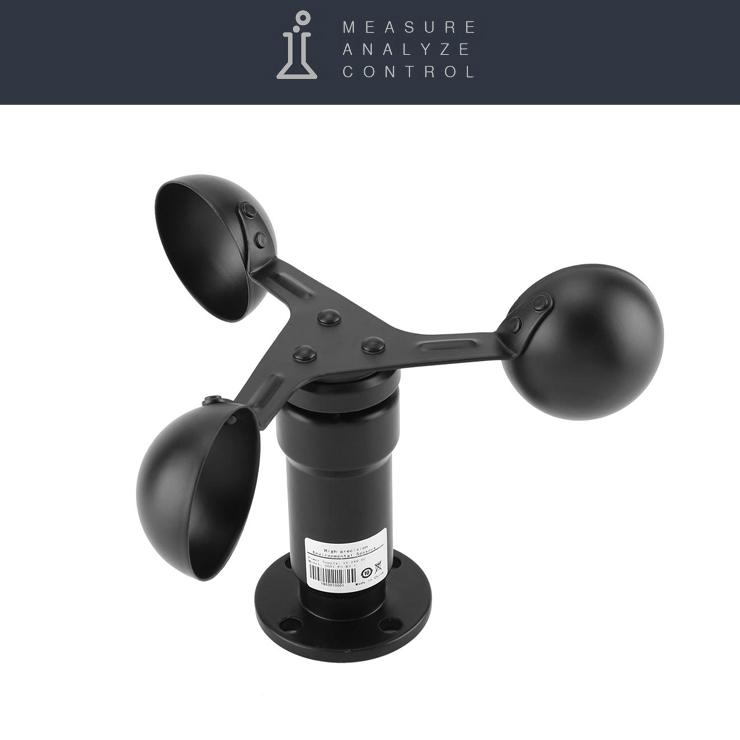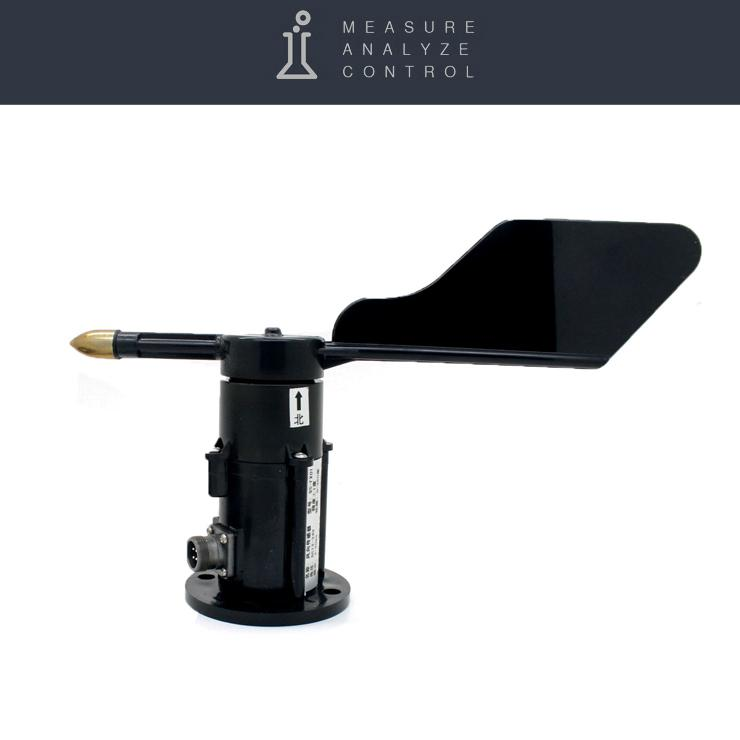The Wind Speed and Wind Direction sensors can be connected to the universal D105 meter.
Dashboard with examples widgets of monitoring wind speed and direction below »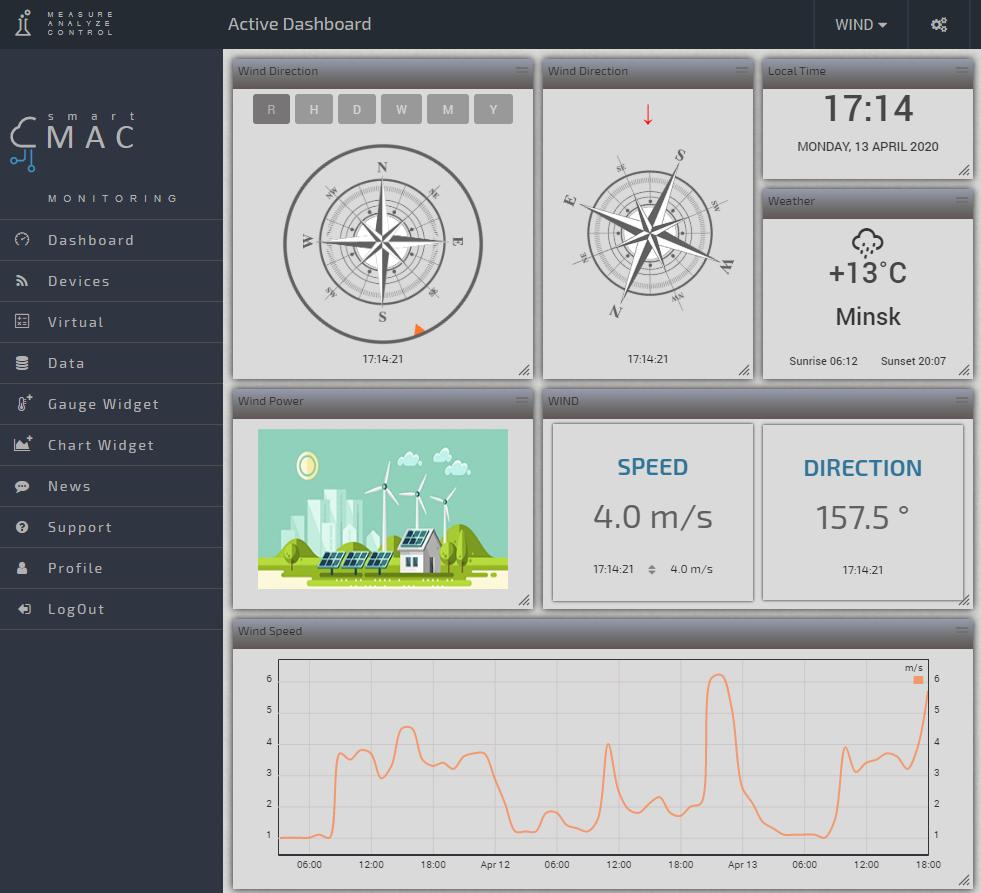### 1. With the digital output via RS485 interface

Wind speed and direction sensors transmit information on the RS485 bus, this bus is notable for stable operation over long distances.

Wind sensors are connected to the RS485 input of the smart meter smart-MAIC D105 G2.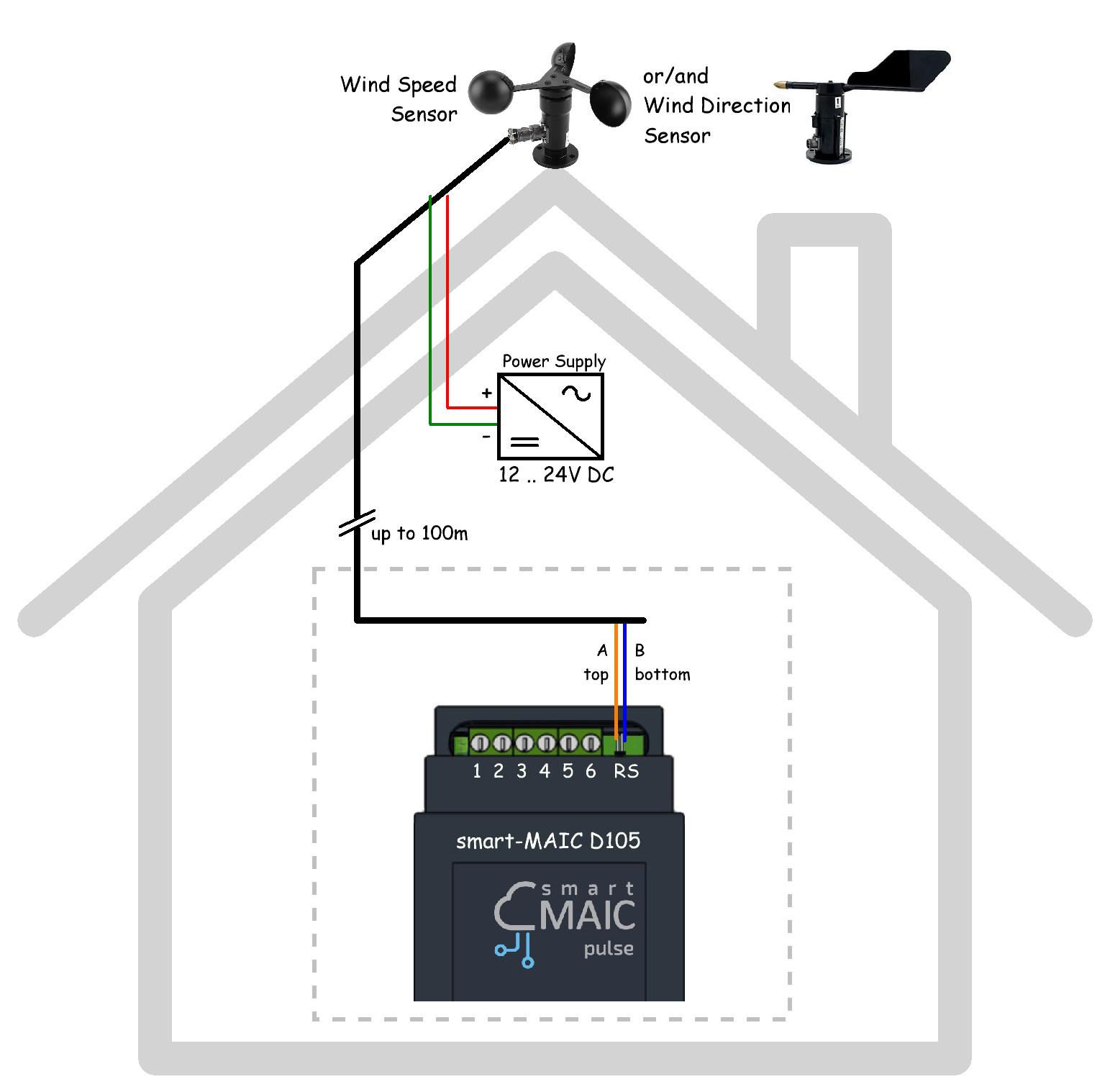In the device settings, in the "Counter Config" menu in the "Setting up the RS485 interface" section, select the type of wind sensor: SPEED and/or DIRECTION. After saving the settings, the device will search for connected sensors.

Data from the wind speed sensor is always stored in parameter [T4].

Data from the wind direction sensor is always stored in parameter [T5].

For the wind speed sensor, the data will be in meters per second.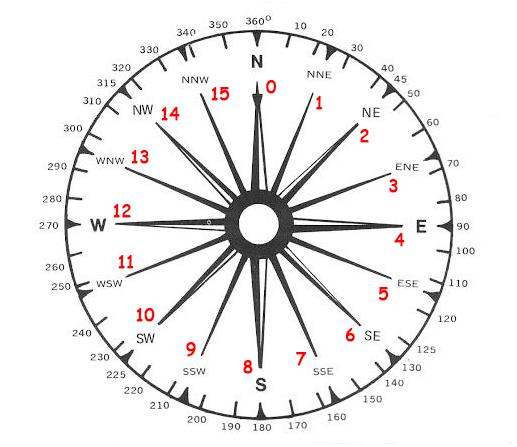For the wind direction sensor, the data will contain a number from 0 to 15, i.e. the sensor has 16 graduations depending on the direction angle in the measuring range 0 .. 360 °. Where 0 corresponds to 0 degrees of the North direction, and each step is to 360 ° / 16 = 22.5 °.
It is necessary to position the sensor North by the mark on the sensor. If it absent, connect the sensor, find the position at which the value is "0" and mark the North yourself.

In the widget settings, in the section "Arithmetic operation", select multiply by 22.5 and get the angle for the direction of the wind.

If you want to display the direction of the wind in the symbols N / NE / E / SE / S, etc. select the function f (x) in the section Arithmetic operation:

`d.T5>13 ? 'NW' :d.T5>11 ? 'W' :d.T5>9 ? 'SW' :d.T5>7 ? 'S' :d.T5>5 ? 'SE' :d.T5>3 ? 'E' :d.T5>1 ? 'NE' : 'N';`

Where d.T5 is the corresponding value to which the wind direction sensor is attached in the device settings.
Hope the function is clear and can be supplemented with intermediate values ​​of NNE / ENE / ESE / SSE etc by yourself.

### 2. With Analog output signal 0 .. 5V / 0 .. 10V / etc.

#### Wind Speed ​​sensor - Anemometer with Analog Output

To calculate wind speed, you need to know the range of speed measurement, for example, 0 - 30 m/s and the range of voltage variation at the analog input, for example, 0 - 5V.

Given the linear relationship between wind speed and voltage at the analog output of the sensor, it is easy to calculate the coefficient for converting voltage to speed. To do this, divide the speed measurement range by the voltage measurement range. For our example:

`K = 30 / 5 = 6`

In the widget settings select the [ADC] Analog data and in the "Arithmetic operation" section multiply by 6.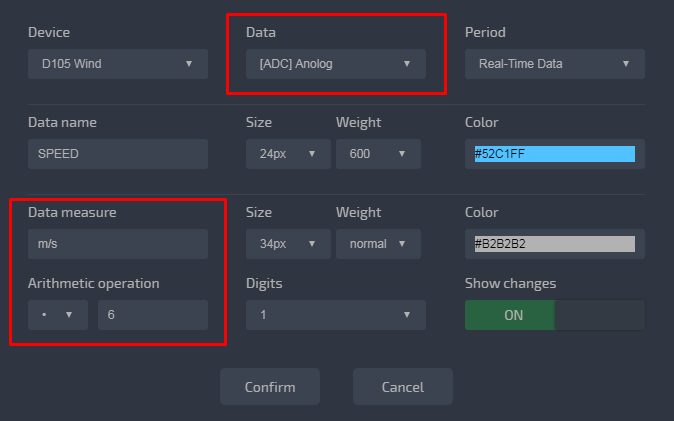Done, now the widget will display the wind speed in meters per second.

#### Wind Direction sensor with Analog Output

To calculate the direction of the wind, you need to know the range of voltage measurement at the analog input, for example, 0-5V, in accordance with the direction. An output voltage of 0V corresponds to 0 degrees North. And it is necessary to position the sensor North by the mark on the sensor. The wind direction sensor has 16 voltage gradations depending on the direction angle in the measurement range 0 .. 360 °.

We can calculate it:

```Voltage step = 5V / 16 = 0.3125V
Direction angle step = 360° / 16 = 22.5°
Wind direction value = ADC / 0.3125 * 22.5 = ADC * (22.5 / 0.3125 ) = ADC * 72```

The coefficient for converting the voltage into the direction angle is 72.

In the widget settings select the [ADC] Analog data and in the "Arithmetic operation" section multiply by 72.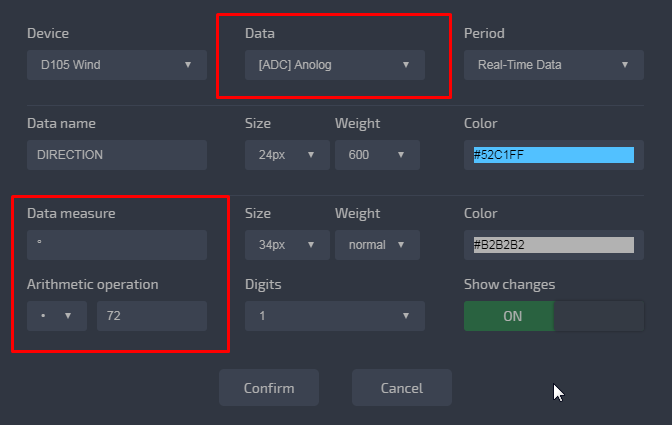Done, now the widget will display the wind direction in the degree 0..360 °.